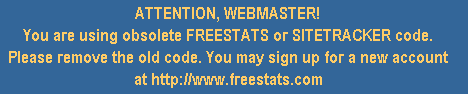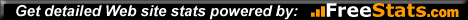# Chaldean Numerology , smChaldean Numerology

• equivalents for the numbers
• 1 - A - I - J - Q - Y - SUN - Sunday
• 2 - B - K - R - MOON - Monday
• 3 - C - G - L - S - JUPITER - Thursday
• 4 - D - M - T - URANUS
• 5 - E - H - N - X - MERCURY - Wednesday
• 6 - U - V - W - VENUS - Friday
• 7 - O - Z - NEPTUNE
• 8 - F - P - SATURN - Saturday
• 9 - MARS & PLUTO - Tuesday"Chaldean Numerology" updated 11-8-98.
Webmeister - GreenHeart Web Page DesignIf you're in the U.S., over 18 years old, and would like to be an independent contractor doing phone readings from the convenience of your home, see our Nostradami site.Chaldean Astrology

Here's a system that seems to have held up about 3,000 years regardless of numerous calendar changes. See The Secret Language of Birthdays by Gary Goldschneider. Also using Chaldean Astrology, The Planetary Hours mentioned in the back of Dell Horoscope magazine use to give Llewellen George's text A-to-Z Horoscope Delineator the credit for their monthly column.Chaldean Numerology

Psuedo-Pythagorean numerology is bogus. Notice that every Pythagorean numerology text avoids any reference of the evolution of the modern English alphabet from the ancient Hebrew alphabet. A validation of Neo-Chaldean numerology is to compare the last name of couples to find the similarity of family trees from the SAME Chaldean value. See Numbers Will Tell by Gerun Moore. See Numerology Made Easy by William Mykian. See Cheiro's Book of Numbers by Cheiro. See Your Numbers of Destiny by Urna and Marlow Gray. If you have an extra copy of Numera by Pan Books, Britain, I'd love to get it from you.The Birth DAY Number

In the Chaldean system, your year of birth and your month of birth are ignored. Only your DAY of birth is used. I was born on the 10th of November 1948, but only the TEN is used for my Birthday number.The Birth NAME Number

The Birthname is a total of two other numbers: the Manifest number and the Soul number. To use Astrological concepts, I would say the Soul number is close to that of the Moon, the Manifest number is close to that of the Ascendant, and the total Birthname number is close to that of the Sun.

Total the consonants for the Manifest number: B, C, D, F, G, H, J, K, L, M, N, P, Q, R, S, T, V, W, X, & Z.

Total the vowels for the Soul number: A, E, I, O, U, & Y.

Andrew Homer Pahn is on my birth certificate. My Manifest number is 5,4,2,6 +5,4,2 +8,5,5 =46 =10 =ONE (Sun). My Soul number is 1,5 +7,5 +1 =19 =10 =ONE (Sun).

But, before you add the numbers of two words, you first reduce the number of that word to a single digit. The 23 of Andrew becomes 5. The 23 of Homer becomes 5. The 19 of Pahn becomes 1. So, my total Birthname number is 11 (Moon).Why Chaldean Numerology?

It makes NO damn logic. When I first studied Astrology, it seemed so irrational, but I honestly studied it so as to debunk it with integrity.

28 years later, I'm still trying to disprove it. But not with much success. Integrity is such a drag.

At least one can imagine some validation to Emerson's comment that "Astrology is Astronomy brought down to Earth and applied to the affairs of Man." But, WHAT association can anyone attach to Numerology? NONE.

But to state that Numerology doesn't work is another conversation.

I'll jump ahead, a bit, and state that it depends WHICH Numerology you're talking about.

I gave Pythagorean Numerology a valiant effort for one full year. Nada.

So, I used my Pythagorean texts only as paperwieghts.

And a year went by.

Then I discovered another Numerology system - Chaldean.

Schazaam. Hot damn. Bingo.

Chaldean Numerology WORKS.

The Chaldean system is in sync with the evolution of the alphabet. The psuedo-Pythagorean system does not.

The Chaldean system holds up to my emperical observations. The psuedo-Pythagorean system does not.

I found that in the Chaldean system, that a wife's maiden name often numerologically matches her husband's last name. If his last name does change her numbers, then I can validly describe the changes she experienced. I can't do that with the psuedo-Pythagorean system.

Why do I call Pythagorean Numerology psuedo-Pythagorean? Because it hardly has ANYTHING to do with Pythagoras. If you prove yourself the scholar and study the evolution of the alphabet, you'll find what I'm talking about. If you aren't a scholar to make a valiant effort, then your opinion doesn't matter anyways.Press the Button to Submit Your Site to 500+ Search Engines & Directories World-Wide!Name Changes

Most people are use to women changing their name when they use their husband's last name. Then, again when they divorce and choose to use their maiden name, again.

Coming from a broken home, I too have had name changes. (The discrimination regarding a man known for having a name change is quite remarkable.) When I was 7 years old, my father killed himself. My mother went to court to have her name changed back to her maiden name, Hacker, and she had the last name of her three children changed, also. So, in 1956, I became Andrew Homer Hacker.

So, while the Numerology of my Birthname, of 1948, will ALWAYS be valid, I acquired an overlay influence in 1956. For you Astrologers, I'll use the example when your Progressed Sun ingresses into the next Zodiac sign.

My new Manifest number became 5,4,2,6 +5,4,2 +5,3,2,2 =40 =4 (Uranus). My new Soul number became 1,5 +7,5 +1,5 =24 =6 (Venus).

To reduce the numbers of Andrew Homer Hacker becomes 5 +5 + 9 =19. (Sun).Power Numbers

The two master numbers in Chaldean numerology are 23 and 19.Compatible Numbers

1 (Sun) and 4 (Uranus) are similar yang numbers. 2 (Moon) and 7 (Neptune) are similar yin numbers. 1 or 4 are compatible with 2 or 7. When you put the nine single numbers into a 3x3 grid, you get these three columns that supposedly reveal some comraderie: 1-4-7, 2-5-8, and 3-6-9. 5, being in the middle of the 3x3 grid, gets along well with the other numbers except for 8. Notice, that when you have Manifest and Soul numbers of 3 and 6, that you'll get the 9, which all three are in that right column of the 3x3 grid.To find the best bargains on the best Numerology texts, go to myFirst Names

AARON - 7+9=16
AGATHA - 12+3=15
AGNES - 11+6=17
ALBERT - 11+6=17
ALBERTA - 11+7=18
ALAN - 8+2=10
ALBERT - 11+6=17
ALLAN - 11+2=13
ALLEN - 11+6=17
ANDREW - 17+6=23
ANN - 10+1=11
ANTHONY - 19+9=28
ANTON - 14+8=22
ARTHUR - 13+7=20
AXEL - 8+6=14BARBARA - 8+3=11
BARRY - 6+2=8
BART - 8+1=9
BASIL - 8+2=10
BERNARD - 11+6=17
BERNICE - 12+11=23
BERTHA - 13+6=19
BRIAN - 9+2=11
BYRON - 9+8=17CARLOS - 11+8=19
CAROL - 8+8=16
CAROLE - 8+13=21
CATHERINE - 19+12=31
CELESTE - 13+15=28
CHARLOTTE - 21+13=34
CHELSEA - 14+10=24
CHERYL - 13+6=19
CLARENCE - 16+11=27
CLAY - 6+2=8
CLYDE - 10+6=16
CONSTANCE - 23+13=36
CYNTHIA - 17+3=20
CYRIL - 8+2=10
CYRUS - 8+7=15DALE - 7+6=13
DAPHNE - 22+6=28
DARIA - 6+3=9
DAVID - 14+2=16
DENISE - 12+11=23
DEXTER - 15+10=25
DIANA - 9+3=12
DIANE - 9+7=16
DONALD - 16+8=24
DONNA - 14+8=22
DOROTHY - 15+15=30EDUARD - 10+12=22
EDUARDO - 10+19=29
EDWARD - 16+6=22
ELEANOR - 10+18=28
EMMA - 8+6=14
ERIC - 5+6=11
ERIK - 4+6=10
ERMA - 6+6=12
EUGENE - 8+21=29
EVAN - 11+6=17FELECIA - 14+12=26
FELIX - 16+6=22
FERDINAND - 28+7=35
FERNANDO - 24+13=37
FLETCHER - 25+10=35
FLOYD - 15+8=23
FRANCO - 18+8=26
FRANK - 17+1=18
FREDERICK - 21+11=32GARY - 5+2=7
GAVIN - 14+2=16
GEORGE - 8+17=25
GERALD - 12+6=18
GERALDO - 12+13=25
GERTRUDE - 15+16=31
GINGER - 16+6=22
GLEN - 11+5=16
GLENDA - 15+6=21
GOLDA - 10+8=18
GORDON - 14+14=28
GREGORY - 10+12=22
GUNTHER - 19+11=30HAL - 8+1=9
HANS - 13+1=14
HAROLD - 14+8=22
HARRIET - 13+7=20
HEATH - 14+6=20
HEATHER - 16+11=27
HECTOR - 14+12=26
HEIDI - 9+7=16
HELEN - 13+10=23
HELGA - 10+6=16
HERBERT - 15+10=25
HERMAN - 16+6=22
HOLLY - 11+8=19
HOMER - 11+12=23
HOPE - 13+12=25INGA - 8+2=10
ISAAC - 6+3=9
ISIAH - 8+3=11JAIME - 5+7=12
JAMES - 8+6=14
JANE - 6+6=12
JANET - 10+6=16
JANICE - 9+7=16
JAY - 1+2=3
JEAN - 6+6=12
JENNIFER - 21+11=32
JEREMY - 7+11=18
JESUS - 7+11=18
JETHRO - 12+12=24
JOHN - 10+7=17
JONATHAN - 20+9=29
JOSE - 4+12=16
JOSEPH - 17+12=29
JOSHUA - 9+14=23
JOY - 1+8=9
JOYCE - 4+13=17
JUDITH - 14+8=22KAREN - 9+6=15
KEITH - 11+6=17
KENNETH - 21+10=31
KERRY - 6+6=12
KIM - 6+1=7
KIMBERLY - 13+7=20LAMAR - 9+2=11
LEE - 3+10=13
LESLIE - 9+11=20
LISL - 9+1=10
LLOYD - 10+8=18
LOIS - 6+8=14
LORI - 5+8=13
LOUIS - 6+14=20
LOUISE - 6+19=25
LUCILLE - 12+12=24
LUIS - 6+7=13
LUISA - 6+8=14MANDY - 13+2=15
MARCELLA - 15+7=22
MARGARET - 15+7=22
MARIA - 6+3=9
MARISOL - 12+9=21
MARTHA - 15+2=17
MARVIN - 17+2=19
MARY - 6+2=8
MATILDA - 15+3=18
MAURY - 6+8=14
MAYME - 8+7=15
MELVIN - 18+6=24
MICHAEL - 15+7=22NANCY - 13+2=15
NATASSIA - 15+4=19
NEAL - 8+6=14
NOEL - 8+12=20
NORMAN - 16+8=24

OSCAR - 8+8=16PATRICIA - 17+4=21
PATRICK - 19+2=21
PAUL - 11+7=18
PAULA - 11+8=19
PAULINE - 16+13=29
PEDRO - 14+12=26
PHYLLIS - 22+2=24
PIERCE - 13+11=24RAMON - 11+8=19
RANDALL - 17+2=19
RHODA - 11+8=19
ROBERT - 10+12=22
ROBERTA - 10+13=23
ROCHELLE - 16+17=33
RODNEY - 11+12=23
ROGER - 7+12=19
ROSA - 5+8=13
ROSALIND - 17+9=26
ROSE - 5+12=17
RUBY - 4+7=11
RUDOLPH - 22+13=35
RUPERT - 16+11=27
RUSSELL - 14+11=25
RUTH - 11+6=17SAMANTHA - 21+3=24
SANDRA - 14+2=16
SEYMOUR - 9+18=27
SEAN - 8+6=14
SHANNON - 23+8=31
SHARON - 15+8=23
SHAWN - 19+1=20
STANLEY - 15+7=22
STEPHANIE - 25+12=37
STEPHEN - 25+10=35
STEVEN - 18+10=28
STEWART - 19+6=25
STUART - 13+7=20
SUSAN - 11+7=18
SUZANNE - 20+12=32
SYDNEY - 12+7=19TAMARA - 10+3=13
TERRY - 8+6=14
TABITHA - 15+3=18
TERRENCE - 16+15=31
TERRY - 8+6=14
TIMOTHY - 17+9=26
TINKA - 11+2=13
TRENT - 15+5=20
TRICIA - 9+3=12
TRISHA - 14+2=16VALERIE - 11+12=23
VERONICA - 16+14=30
VICTOR - 15+8=23
VICTORIA - 15+10=25
VIRGINIA - 16+4=20
VIVIAN - 17+3=20

This page has been visitedtimes.WANDA - 15+2=17
WARREN - 15+6=21
WAYNE - 11+7=18
WENDY - 15+6=21
WILLIAM - 16+3=19
WILMA - 13+2=15

YANCY - 9+2=11

ZOE - 7+12=191 - ONE

10 - TEN

Andrew Homer (1/9).

19 - NINETEEN

Andrew Homer Hacker (4/6), Ark Bazaar (6/4), Bahaifa (6/4).

28 - TWENTY-EIGHT

37 - THIRTY-SEVEN

GreenHeart (3/7), Wiseworld (6/4).

46 - FORTY-SIX

55 - FIFTY-FIVE2 - TWO

11 - ELEVEN

20 - TWENTY

29 - TWENTY-NINE

38 - THIRTY-EIGHT3 - THREE

12 - TWELVE

21 - TWENTY-ONE

30 - THIRTY

39 - THIRTY-NINE4 - FOUR

13 - THIRTEEN

22 - TWENTY-TWO

31 - THIRTY-ONE

40 - FORTY5 - FIVE

14 - FOURTEEN

23 - TWENTY-THREE

Andrew (8/6), Homer (2/3).

32 - THIRTY-TWO

41 - FORTY-ONE6 - SIX

15 - FIFTEEN

24 - TWENTY-FOUR

33 - THIRTY-THREE

42 - FORTY-TWO7 - SEVEN

16 - SIXTEEN

25 - TWENTY-FIVE

34 - THIRTY-FOUR

43 - FORTY-THREE8 - EIGHT

17 - SEVENTEEN

26 - TWENTY-SIX

35 - THIRTY-FIVE

44 - FORTY-FOUR9 - NINE

18 - EIGHTEEN

27 - TWENTY-SEVEN

StarHeart (2/7).

36 - THIRTY-SIX

45 - FORTY-FIVEAstrology Odyssey MESSAGE BOARDAndrew Homer's sites to beholdEmail: Chaldean_Numerology@bigfoot.com